# There's No End to It -- Matlab Code Plots Frequency Response above the Unit Circle

Reference  has some 3D plots of frequency response magnitude above the unit circle in the Z-plane.  I liked them enough that I wrote a Matlab function to plot the response of any digital filter this way.  I’m not sure how useful these plots are, but they’re fun to look at. The Matlab code is listed in the Appendix.

This post is available in PDF format for easy printing

## plotfil3d

Purpose             Plot frequency response around the unit circle above the Z-plane.

Syntax

plotfil3d(b,a,N)

plotfil3d(b,a,N,az)

Description

plotfil3d(b,a,N) plots the frequency response magnitude of a digital filter as a 3D curve above the unit circle in the Z-plane.  Vertical axis units are dB.  The frequency response is computed using the Matlab function freqz(b,a,N,’whole’) as

$$H(z) = \frac{b(1)+b(2)z^{-1}+b(3)z^{-2}+...}{a(1)+a(2)z^{-1}+a(3)z^{-2}+...}$$

where b and a are the filter coefficient vectors and z = e.  The response is computed for N values of ω equally spaced over 0 to 2π.

To plot the response for an FIR filter, set a =  1.  For efficient computation, N should be chosen as a power of 2.

plotfil3d(b,a,N,az) plots the frequency response as above, except the plot viewing azimuth in degrees is determined by az.

### Example 1

Plot the magnitude response of an FIR lowpass filter with cutoff frequency = 0.15*fs.

Compute filter coefficients:

fnorm= .3;                 % fnorm = fc/(fs/2)
b= fir1(30,fnorm);         % window-based FIR coeffs, order = 3


Create a conventional response plot in two dimensions:

N= 512;
[h,f]= freqz(b,1,N,'whole',1);   % freq response
H= 20*log10(abs(h));             % dB freq response
plot(f,H),grid
axis([0 1 -80 5]),xlabel('f/fs'),ylabel('dB')


The 2D plot is shown in Figure 1.  Now plot in three dimensions:

plotfil3d(b,1,N);     % plot with default viewing azimuth


The resulting response, shown in Figure 2,  is basically Figure 1 formed into a cylinder.   Note that frequency increases in the counter-clockwise direction around the unit circle, so the frequencies from 0 to fs/2 are on the left  (although this is not apparent because the response is symmetrical).  We can also look at the response from another angle.  Using an azimuth of 120 degrees places the frequencies from 0 to fs/2 on the right (Figure 3):

az= 120;                 % degrees azimuth for viewing plot
plotfil3d(b,1,N,az);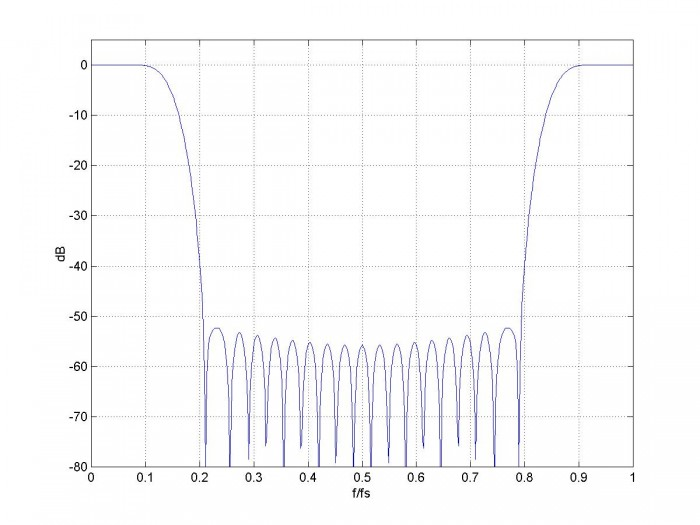Figure 1.  Conventional Plot of magnitude response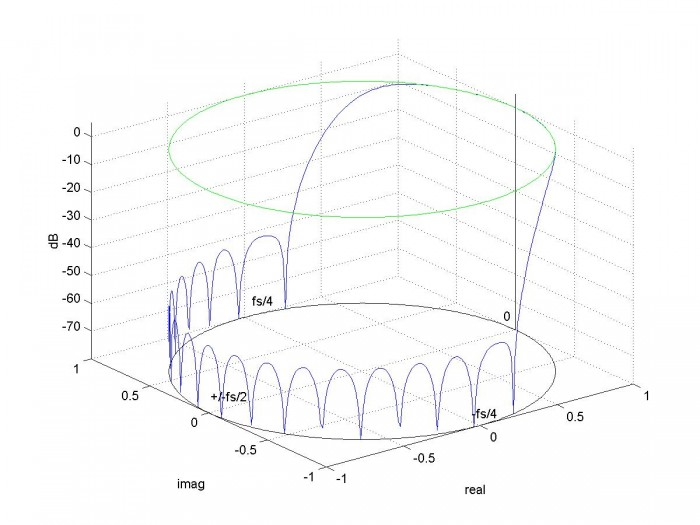Figure 2.  3D Plot of magnitude response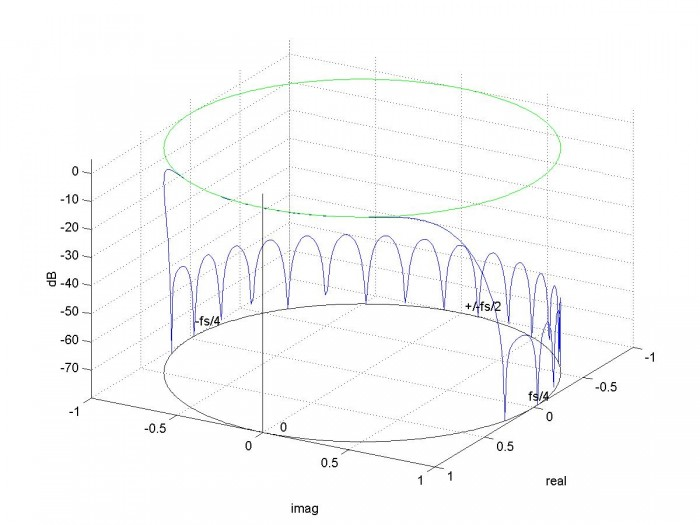Figure 3.  3D plot with azimuth = 120 degrees

### Example 2

Plot the magnitude response of an FIR bandpass filter.  Let sample frequency = 200 Hz, lower stopband = 0 to 25 Hz,  passband = 37 to 43 Hz, upper stopband = 55 to 100 Hz.

Nfil= 62;                     % filter order
f= [0 25 37 43 55 100]/100;   % define stopband and passband freqs
a = [0 0 1 1 0 0];            % bpf goal function
b= firpm(nfil,f,a);           % synthesis using Parks-McClellan algorithm
N= 512;
plotfil3d(b,1,N)


The response magnitude is shown in Figure 4.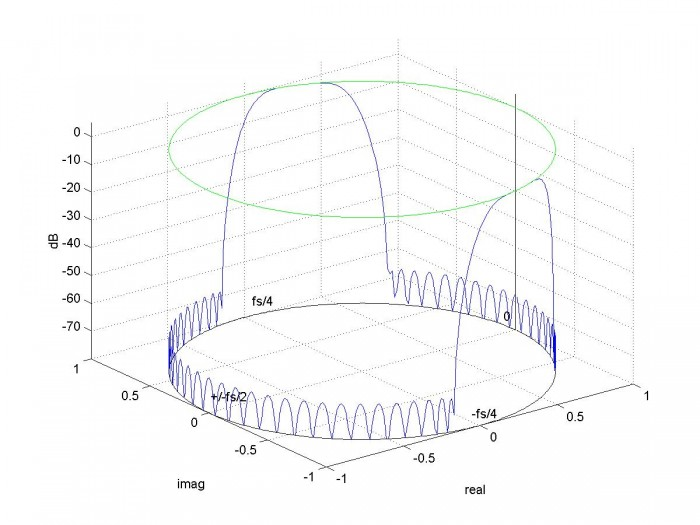Figure 4.  Magnitude response of bandpass FIR filter

### Example 3

Plot the magnitude response of a 5th order Butterworth IIR filter with cutoff frequency of 0.08*fs.  Plot the filter’s poles and zeros in the Z-plane.

fnorm= .16;                   % fnorm = fc/(fs/2)
[b,a]= butter(5,fnorm);       % synthesize 5th order Butterworth IIR filter
N= 256;
plotfil3d(b,a,N);
q= roots(b)                   % zeros of H(z)
p= roots(a)                   % poles of H(z)
plot3(real(p),imag(p),-80*ones(1,5),'xr')
plot3(real(q),imag(q),-80*ones(1,5),'or')


There are 5 zeros, all near -1 + j0:

q =
-1.0008
-1.0003 + 0.0008i
-1.0003 - 0.0008i
-0.9993 + 0.0005i
-0.9993 - 0.0005i


The 5 poles are:

p =
0.7628 + 0.3988i
0.7628 - 0.3988i
0.6306 + 0.2038i
0.6306 - 0.2038i
0.5914


The response plot with poles and zeros is shown in Figure 5.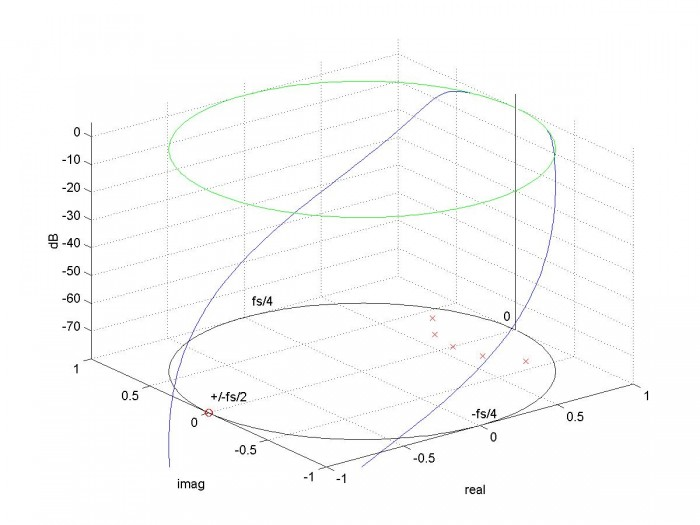Figure 5.  Magnitude response of 5th order Butterworth LP filter, with poles and zeros shown in the  Z-plane.

Reference

1.  Lyons, Richard, Understanding Digital Signal Processing 2nd Ed., Prentice Hall, 2011, p 288.

Appendix    Matlab Function plotfil3d

% plotfil3d.m     10/17/17  revised 11/13/17  Neil Robertson
% Plot Magnitude Response above unit circle in the z-plane
% note w (omega) is defined as w= 2*pi*f/fs = 2*pi*k/N (radians).
% For f= fs/2, w = pi
function plotfil3d(b,a,N,az);
clf
if nargin < 4
az= -37.5;                    % degrees  default viewing azimuth
end
h = freqz(b,a,N,'whole');        % freq response from 0 to 2*pi
H= 20*log10(abs(h)+eps);   % dB freq response (eps= 2E-16 prevents log of 0)
% unit circle in z-plane
k= 0:N-1;                        % frequency index
z= exp(j*w);                     % unit circle
% plot frequency response and unit circles
H(H<-80)= -80;                   % limit min value of H to -80 dB
plot3(real(z),imag(z),H),grid
view(az,30)                      % use az (degrees) to set viewing azimuth
top= max(H);
hold on
plot3([1 1],[0 0],[top-80 top+5],'k')           % z axis
plot3(real(z),imag(z),(top-80)*ones(1,N),'k')   % unit circle at max(H)-80 dB
plot3(real(z),imag(z),top*ones(1,N),'g')        % unit circle at max(H) dB
xlabel('real'),ylabel('imag'),zlabel('dB')
axis([-1 1 -1 1 top-80 top+5])
% frequency labels
text(1,.1,top-77,'0')
text(.05, 1,top-75,'fs/4')
text(-.95,.05,top-76,'+/-fs/2')
text(-.05, -1,top-75,'-fs/4')


[ - ]
Comment by October 24, 2017Oh come on Neil, you seem bored in your retirement. Many of us can hardly manage the 2D plot. Thanks anyway and at least it proves that 2D is subset of 3D

[ - ]
Comment by October 24, 2017Kaz,

Yes, I may be getting to the bottom of my shallow well of DSP knowledge!

Neil

[ - ]
Comment by November 13, 2017Hi Neil.   Those plots are neat!

[ - ]
Comment by November 13, 2017Rick,

Thanks, I'll add you to the list of admirers (so far that's you and my mother).

For some reason, I just find them to be mesmerizing.

Neil

[ - ]
Comment by November 13, 2017Regarding your 'plotfil3d()' function, you might try thresholding the computed 'H' sequence using:

Threshold = -80;  % dB threshold level for specmag plots
H(H<Threshold) = Threshold;  % Min dB plot level)>

to see if that makes your plots look even prettier.  (Of course you can change that -80 value to some other value.)

Here's your Figure 4 using the -80 (dB) value: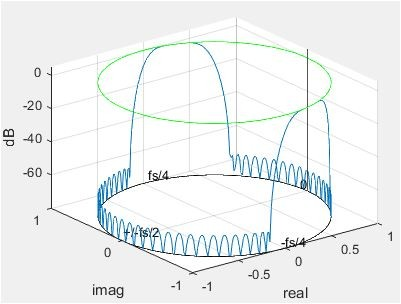[ - ]
Comment by November 13, 2017Rick,

Thanks for the suggestion.  I updated the post to include this feature.

regards,

Neil

[ - ]
Comment by January 5, 2018The zplane function could be helpful for visualizing (aspects of) these things as well:

Wn = 300/500;

[b,a] = butter(3,Wn,'high');

zplane(b, a);

[ - ]
Comment by January 5, 2018My previous post looks like this: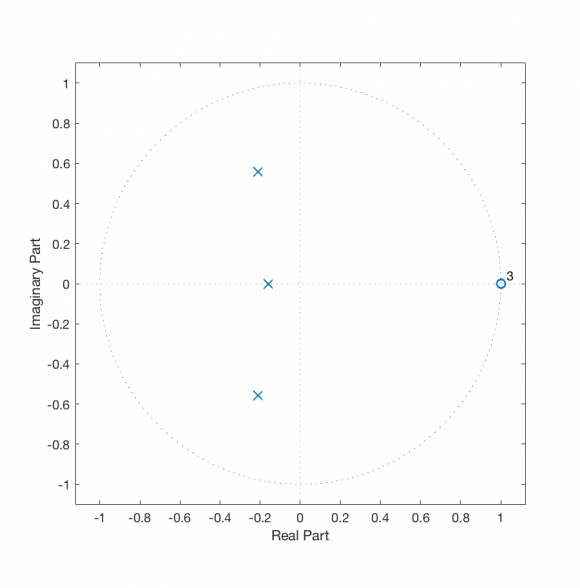I suppose you could do something ala this as well (my z-plane comprehension is getting rusty at this point):

Wn = 300/500;
N = 3;
[b,a] = butter(3,Wn,'high');
[X, Y] = meshgrid(-2:.01:2, -2:.01:2);
Z = X+1j*Y;
Zev = sum(shiftdim(b, -1) .* Z.^shiftdim(-1:-1:-(N+1), -1), 3) ./ ...
sum(shiftdim(a, -1) .* Z.^shiftdim(-1:-1:-(N+1), -1), 3);[ - ]
Comment by January 5, 2018Nifty, thanks.

Neil

To post reply to a comment, click on the 'reply' button attached to each comment. To post a new comment (not a reply to a comment) check out the 'Write a Comment' tab at the top of the comments.